# Euler-Frobenius polynomials

(diff) ← Older revision | Latest revision (diff) | Newer revision → (diff)

The Euler–Frobenius polynomialsof degreeare characterized by the Frobenius reciprocal identity ([a1], [a2], [a3])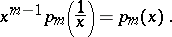Thus,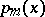is invariant under the reflection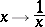of the indeterminate. The best way to implement an invariance of this kind is to look for an appropriate space with which the Euler–Frobenius polynomialsare attached in a spectral geometric way.

So, letdenote a symplectic vector space of dimension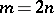(cf. also Symplectic space). Then the characteristic polynomial of each symplectic automorphism ofis an Euler–Frobenius polynomialof odd degree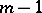.

The proof follows from the fact that the determinant of each symplectic automorphismofequals, so that there is a natural imbeddingThus,preserves the symplectic volume spanned byvectors of the vector space.

A consequence is that each eigenvalue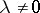of a symplectic endomorphismofhaving multiplicitygives rise to a reciprocal eigenvalueof the same multiplicity.

In view of the self-reciprocal eigenvalue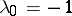of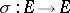for even, of course, spectral theory suggests a complex contour integral representation of the Euler–Frobenius polynomials, as follows.

Letdenote a complex number such that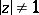. Letdenote a path in the complex planewhich forms the boundary of a closed vertical strip in the open right or left half-plane ofaccording as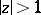or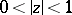, respectively. Let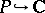be oriented so that its topological index satisfies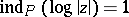. Then, for each integer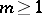, the complex contour integral representation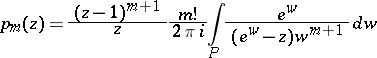holds.

The proof follows from the expansionwith strictly positive integer coefficients, where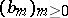denote the basis spline functions (cf. [a3] and also Spline).

A consequence is that the Euler–Frobenius polynomials provide the coefficients of the local power series expansion of the function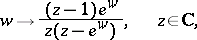which is meromorphic on the complex plane.

The Euler–Frobenius polynomials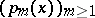satisfy the three-term recurrence relation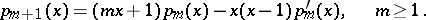A direct proof follows from the complex contour integral representations of the derivatives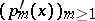, which can be derived from the complex contour integral representation given above for the Euler–Frobenius polynomials.

The preceding recurrence relation opens a simple way to calculate the coefficients of the Euler–Frobenius polynomials([a1], [a3]).

How to Cite This Entry:
Euler-Frobenius polynomials. Encyclopedia of Mathematics. URL: http://encyclopediaofmath.org/index.php?title=Euler-Frobenius_polynomials&oldid=17228
This article was adapted from an original article by Walter Schempp (originator), which appeared in Encyclopedia of Mathematics - ISBN 1402006098. See original article